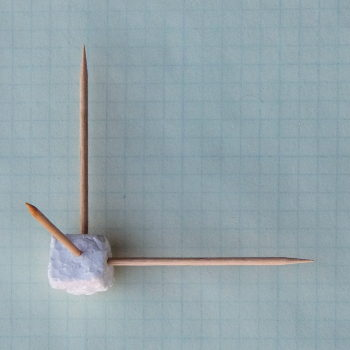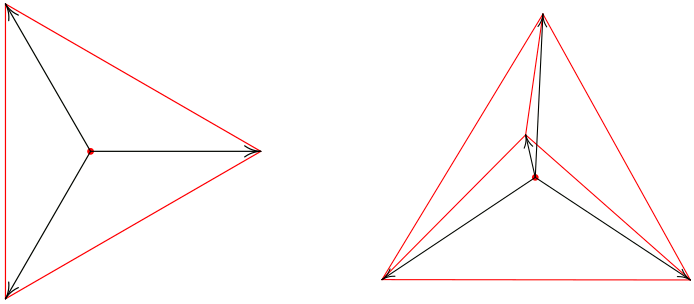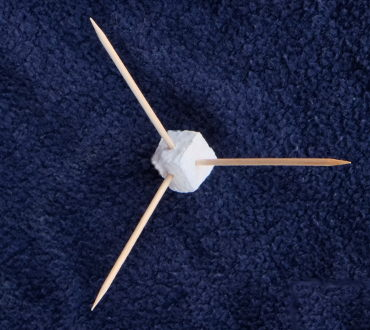Cartesian coordinates and simplicial coordinates

 Cartesian coordinates use a set of mutually orthogonal unit basis vectors to span a space. In the photo to the right, the familiar 3‑D Cartesian unit vectors ı⃗, ȷ⃗, k⃗ are modeled with toothpicks stuck into a styrofoam origin. These vectors satisfy the definition of a basis for 3‑space:they are linearly independent(there's no way to express any of the basis vectors as a linear combination of the others)they span 3‑space(any point in 3‑space can be expressed as a linear combination of the basis vectors) Together, those two properties provide that any point in 3‑space has unique Cartesian coordinates (x,y,z).Simplicial coordinates define unit vectors pointing from the center of a simplex to its vertices. This discussion considers simplicial coordinates based on regular simplexes. To map a plane, simplicial coordinates use three unit vectors pointing toward the vertices of an equilateral triangle. To map space, simplicial coordinates use four unit vectors pointing toward the vertices of a regular tetrahedron. Planar and spatial simplicial unit vectors (for triangular and tetrahedral coordinates, respectively) are the black arrows in these illustrations:Simplicial coordinates generalize to higher dimensions and in each case use one more coordinate than Cartesian coordinates do.

The unit vectors defined in simplicial coordinates are not linearly independent and thus not a basis by the conventional linear algebra definition.

Whereas Cartesian coordinates for a point are unique, simplicial coordinates are not. Any triple of triangular coordinates (a,b,c) is a member of an infinite equivalence class of triples (a+m,b+m,c+m) all referring to the same point (where m is any real number). Similarly, any 4‑tuple (a,b,c,d) of tetrahedral coordinates is a member of an equivalence class of 4‑tuples (a+m,b+m,c+m,d+m).

Non‑uniqueness is hardly unprecedented in coordinate systems. Polar coordinates (r,θ) belong to an equivalence class of coordinates (r,θ+2πn) all referring to the same point (where n is any integer).

The reader may be wondering what simplicial coordinates are good for. I'll mention some applications later but this discussion is primarily about the form and character of simplicial coordinates which are of interest in their own right.

Let's consider calculations. Using Cartesian coordinates, distance between two points is readily calculated by a Pythagorean formula. How would you calculate distance between points at triangular coordinates (a1,b1,c1) and (a2,b2,c2)? How would you calculate the dot product of two vectors in a plane from their triangular coordinates? How would these and other calculations be done using tetrahedral spatial coordinates?

Simplicial coordinates seem to have a notably different flavor than Cartesian coordinates. Whereas Cartesian unit basis vectors are at 90° to one another, tetrahedral coordinate unit vectors are at about 109.47°. But the two types of system are more related than they might seem at first blush.

Consider the plane defined by the equation x+y+z=0 in Cartesian 3‑D coordinates. This plane has normal vector <1,1,1>, includes the origin, and is parallel to the plane that includes the points (1,0,0), (0,1,0), and (0,0,1).

The orthogonal projection of any point with Cartesian coordinates (x,y,z) onto the plane x+y+z=0 can be found by subtracting (x+y+z)/3 from all three coordinates. E.g., the point at (1,4,4) projects to (‑2,1,1). A vector from (1,4,4) to its projection at (-2,1,1) is orthogonal (i.e., normal) to the plane.

The set of points which project to any given point (x,y,z) on the plane x+y+z=0 is an equivalence class. The points' coordinates are given by (x+m,y+m,z+m) where m is any real number. This is the same computational form seen in the equivalence class of triangular coordinates described earlier.

The relationship between Cartesian 3-D coordinates and planar triangular coordinates can be appreciated visually. Viewed from a certain perspective—e.g., with one's eye at (10,10,10)—the three Cartesian unit basis vectors in space look like the three unit vectors of planar triangular coordinates. The photo below is an oblique view of the same toothpick model of Cartesian unit vectors seen in a photo at the top of this page.This is a useful correspondence: planar simplicial coordinates work like Cartesian 3‑space coordinates projected onto the plane x+y+z=0. To perform any desired calculation with triangular coordinates (a,b,c): reconsider them as Cartesian coordinates (x,y,z), project them onto the plane x+y+z=0, and use established methods for calculating with Cartesian coordinates. The projection step is as described earlier: subtract (x+y+z)/3 from all three coordinates. The results of some calculations will require scaling, as the Cartesian basis vectors no longer have unity length after projection.

An analogous correspondence exists between tetrahedral coordinates and Cartesian 4‑space coordinates. The correspondence can be applied in a strictly formal manner, i.e. it requires no superhuman ability to visualize higher-dimensional spaces. To calculate with tetrahedral coordinates (a,b,c,d): normalize by subtracting (a+b+c+d)/4 from all four coordinates, then calcuate by considering the resulting coordinates to be Cartesian 4‑D coordinates projected into a normalized 3‑space. Again, scaling will be required for some calculations. Sample code for calculating with tetrahedral (also known as "quadray") coordinates is at http://minortriad.com/quadray.html

A word is in order as to why this correspondence works in spatial and higher dimensions. It's easy enough to see how Cartesian 3‑D basis vectors project to triangular coordinate vectors in a plane, but how can we know that Cartesian 4‑D basis vectors indeed project to the vertices of a regular tetrahedron?

Cartesian basis vectors in n dimensions have coordinates (1,0,...,0,0), (0,1,...0,0), ... (0,0,...,1,0), (0,0,...,0,1). Each vector has a single 1 coordinate and all the rest 0, and the 1 coordinate is in a different place in each vector.

To project the basis vectors, subtract 1/n from each coordinate. Each 0 coordinate becomes ‑1/n and each 1 coordinate becomes (n‑1)/n. These projected vectors all have the same length, sqrt((n‑1)/n). The dot product of any pair of projected vectors is (‑1/n). Thus the angle subtended by any pair of vectors is arccos(‑1/(n‑1)). (I've omitted some steps of algebra here; it's all straightforward.) Together, the uniformity of length and uniformity of subtended angle between pairs suffice to show that the n projected vectors point to vertices of an n‑1 dimensional regular simplex. Note that arccos(-1/3) indeed gives the angle between any pair of tetrahedral coordinate unit vectors (approximately 109.47°).

Regarding applications of simplicial coordinates: they can be normalized to be identical to barycentric coordinates, for which Wikipedia describes several applications. The normalization step to convert simplicial coordinates into barycentrics is similar to the projection step in the discussion above, except that barycentric coordinates sum to 1 rather than to 0. E.g, normalize triangular coordinates (a,b,c) by subtracting (a+b+c1)/3 from each coordinate.

Tetrahedral coordinates also have pedagogical value by virtue of being an alternative to more familiar systems. Just as studying a second language leads to deeper understanding of one's mother tongue, one gains perspective from study of alternate mathematical representations. For discussions on conceptual and philosophical aspects of tetrahedral (also called "quadray") coordinates, see Kirby Urner's http://www.grunch.net/synergetics/quadphil.html

Tom Ace